## Buildings.Fluid.Sensors

Package with sensor models

### Information

Package `Sensors` consists of idealized sensor components that provide variables of a medium as output signals. These signals can be, e.g., further processed with components of the Modelica.Blocks library.

Extends from Modelica.Icons.SensorsPackage (Icon for packages containing sensors).

### Package Content

Name DescriptionUsersGuide User's GuideDensity Ideal one port density sensorDensityTwoPort Ideal two port density sensorEnthalpyFlowRate Ideal enthalphy flow rate sensorEntropyFlowRate Ideal entropy flow rate sensorLatentEnthalpyFlowRate Ideal enthalphy flow rate sensor that outputs the latent enthalpy flow rate onlyMassFlowRate Ideal sensor for mass flow rateMassFraction Ideal one port mass fraction sensor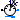MassFractionTwoPort Ideal two port mass fraction sensorPPM Ideal one port trace substances sensor outputting in parts per millionPPMTwoPort Ideal two port trace substances sensor outputting in parts per millionPressure Ideal pressure sensorRelativeHumidity Ideal one port relative humidity sensor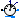RelativeHumidityTwoPort Ideal two port relative humidity sensor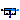RelativePressure Ideal relative pressure sensorRelativeTemperature Ideal relative temperature sensor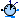SensibleEnthalpyFlowRate Ideal enthalphy flow rate sensor that outputs the sensible enthalpy flow rate only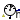SpecificEnthalpy Ideal one port specific enthalpy sensorSpecificEnthalpyTwoPort Ideal two port sensor for the specific enthalpySpecificEntropy Ideal one port specific entropy sensorSpecificEntropyTwoPort Ideal two port sensor for the specific entropy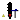Temperature Ideal one port temperature sensorTemperatureTwoPort Ideal two port temperature sensorTemperatureWetBulbTwoPort Ideal wet bulb temperature sensorTraceSubstances Ideal one port trace substances sensorTraceSubstancesTwoPort Ideal two port sensor for trace substance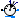Velocity Ideal sensor for flow velocityVolumeFlowRate Ideal sensor for volume flow rateConversions Package with conversions for sensor models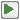Examples Collection of models that illustrate model use and test modelsBaseClasses Package with base classes for Buildings.Fluid.Sensors

##Buildings.Fluid.Sensors.Density

Ideal one port density sensor### Information

This model outputs the density of the fluid connected to its port. The sensor is ideal, i.e. it does not influence the fluid.

To measure density in a duct or pipe, use Buildings.Fluid.Sensors.DensityTwoPort rather than this sensor. Read the Buildings.Fluid.Sensors.UsersGuide prior to using this model to see about potential numerical problems if this sensor is used incorrectly in a system model.

Extends from Buildings.Fluid.Sensors.BaseClasses.PartialAbsoluteSensor (Partial component to model a sensor that measures a potential variable), Modelica.Icons.RotationalSensor (Icon representing a round measurement device).

### Parameters

TypeNameDefaultDescription
replaceable package MediumPartialMediumMedium in the sensor

### Connectors

TypeNameDescription
FluidPort_aport
output RealOutputdDensity in port medium [kg/m3]

### Modelica definition

model Density "Ideal one port density sensor" extends Buildings.Fluid.Sensors.BaseClasses.PartialAbsoluteSensor; extends Modelica.Icons.RotationalSensor; Modelica.Blocks.Interfaces.RealOutput d(final quantity="Density", final unit="kg/m3", min=0) "Density in port medium"; equation d = Medium.density( state=Medium.setState_phX( p=port.p, h=inStream(port.h_outflow), X=inStream(port.Xi_outflow))); end Density;

##Buildings.Fluid.Sensors.DensityTwoPort

Ideal two port density sensor### Information

This model outputs the density of the fluid flowing from `port_a` to `port_b`.

The sensor is ideal, i.e., it does not influence the fluid. If the parameter `tau` is non-zero, then its output is computed using a first order differential equation. Setting `tau=0` is not recommend. See Buildings.Fluid.Sensors.UsersGuide for an explanation.

Extends from Buildings.Fluid.Sensors.BaseClasses.PartialDynamicFlowSensor (Partial component to model sensors that measure flow properties using a dynamic model), Modelica.Icons.RotationalSensor (Icon representing a round measurement device).

### Parameters

TypeNameDefaultDescription
replaceable package MediumPartialMediumMedium in the component
Timetau1Time constant at nominal flow rate (use tau=0 for steady-state sensor, but see user guide for potential problems) [s]
Nominal condition
MassFlowRatem_flow_nominal Nominal mass flow rate, used for regularization near zero flow [kg/s]
Initialization
InitinitTypeModelica.Blocks.Types.Init.I...Type of initialization (InitialState and InitialOutput are identical)
Densityd_startMedium.density(state=Medium....Initial or guess value of output (=state) [kg/m3]
TemperatureT_startMedium.T_defaultTemperature used to compute d_start [K]
Pressurep_startMedium.p_defaultPressure used to compute d_start [Pa]
MassFractionX_start[Medium.nX]Medium.X_defaultMass fraction used to compute d_start 
Assumptions
BooleanallowFlowReversaltrue= false to simplify equations, assuming, but not enforcing, no flow reversal
MassFlowRatem_flow_small1E-4*m_flow_nominalFor bi-directional flow, temperature is regularized in the region |m_flow| < m_flow_small (m_flow_small > 0 required) [kg/s]

### Connectors

TypeNameDescription
FluidPort_aport_aFluid connector a (positive design flow direction is from port_a to port_b)
FluidPort_bport_bFluid connector b (positive design flow direction is from port_a to port_b)
output RealOutputdDensity of the passing fluid [kg/m3]

### Modelica definition

model DensityTwoPort "Ideal two port density sensor" extends Buildings.Fluid.Sensors.BaseClasses.PartialDynamicFlowSensor; extends Modelica.Icons.RotationalSensor; Modelica.Blocks.Interfaces.RealOutput d(final quantity="Density", final unit="kg/m3", min=0) "Density of the passing fluid"; parameter Medium.Density d_start= Medium.density(state=Medium.setState_pTX( p=p_start, T=T_start, X=X_start)) "Initial or guess value of output (=state)"; parameter Modelica.SIunits.Temperature T_start=Medium.T_default "Temperature used to compute d_start"; parameter Modelica.SIunits.Pressure p_start=Medium.p_default "Pressure used to compute d_start"; parameter Modelica.SIunits.MassFraction X_start[Medium.nX]=Medium.X_default "Mass fraction used to compute d_start"; protected Medium.Density dMed(start=d_start) "Medium density to which the sensor is exposed"; Medium.Density d_a_inflow "Density of inflowing fluid at port_a"; Medium.Density d_b_inflow "Density of inflowing fluid at port_b, or rho_a_inflow if uni-directional flow"; initial equation if dynamic then if initType == Modelica.Blocks.Types.Init.SteadyState then der(d) = 0; elseif initType == Modelica.Blocks.Types.Init.InitialState or initType == Modelica.Blocks.Types.Init.InitialOutput then d = d_start; end if; end if; equation if allowFlowReversal then d_a_inflow = Medium.density( state=Medium.setState_phX(p=port_b.p, h=port_b.h_outflow, X=port_b.Xi_outflow)); d_b_inflow = Medium.density( state=Medium.setState_phX(p=port_a.p, h=port_a.h_outflow, X=port_a.Xi_outflow)); dMed = Modelica.Fluid.Utilities.regStep( x=port_a.m_flow, y1=d_a_inflow, y2=d_b_inflow, x_small=m_flow_small); else dMed = Medium.density( state=Medium.setState_phX(p=port_b.p, h=port_b.h_outflow, X=port_b.Xi_outflow)); d_a_inflow = dMed; d_b_inflow = dMed; end if; // Output signal of sensor if dynamic then der(d) = (dMed-d)*k*tauInv; else d = dMed; end if; end DensityTwoPort;

##Buildings.Fluid.Sensors.EnthalpyFlowRate

Ideal enthalphy flow rate sensor### Information

This model outputs the enthalphy flow rate of the medium in the flow between fluid ports. The sensor is ideal, i.e., it does not influence the fluid.

If the parameter `tau` is non-zero, then the measured specific enthalpy hout that is used to compute the enthalpy flow rate Ḣ = ṁ hout is computed using a first order differential equation. See Buildings.Fluid.Sensors.UsersGuide for an explanation.

For a sensor that measures the latent enthalpy flow rate, use Buildings.Fluid.Sensors.LatentEnthalpyFlowRate.

Extends from Buildings.Fluid.Sensors.BaseClasses.PartialDynamicFlowSensor (Partial component to model sensors that measure flow properties using a dynamic model), Modelica.Icons.RotationalSensor (Icon representing a round measurement device).

### Parameters

TypeNameDefaultDescription
replaceable package MediumPartialMediumMedium in the component
Timetau0Time constant at nominal flow rate (use tau=0 for steady-state sensor, but see user guide for potential problems) [s]
Nominal condition
MassFlowRatem_flow_nominal Nominal mass flow rate, used for regularization near zero flow [kg/s]
Initialization
InitinitTypeModelica.Blocks.Types.Init.I...Type of initialization (InitialState and InitialOutput are identical)
SpecificEnthalpyh_out_startMedium.specificEnthalpy_pTX(...Initial or guess value of measured specific enthalpy [J/kg]
Assumptions
BooleanallowFlowReversaltrue= false to simplify equations, assuming, but not enforcing, no flow reversal
MassFlowRatem_flow_small1E-4*m_flow_nominalFor bi-directional flow, temperature is regularized in the region |m_flow| < m_flow_small (m_flow_small > 0 required) [kg/s]

### Connectors

TypeNameDescription
FluidPort_aport_aFluid connector a (positive design flow direction is from port_a to port_b)
FluidPort_bport_bFluid connector b (positive design flow direction is from port_a to port_b)
output RealOutputH_flowEnthalpy flow rate, positive if from port_a to port_b [W]

### Modelica definition

model EnthalpyFlowRate "Ideal enthalphy flow rate sensor" extends Buildings.Fluid.Sensors.BaseClasses.PartialDynamicFlowSensor( tau=0); extends Modelica.Icons.RotationalSensor; Modelica.Blocks.Interfaces.RealOutput H_flow(final unit="W") "Enthalpy flow rate, positive if from port_a to port_b"; parameter Modelica.SIunits.SpecificEnthalpy h_out_start= Medium.specificEnthalpy_pTX( p=Medium.p_default, T=Medium.T_default, X=Medium.X_default) "Initial or guess value of measured specific enthalpy"; protected Modelica.SIunits.SpecificEnthalpy hMed_out(start=h_out_start) "Medium enthalpy to which the sensor is exposed"; Modelica.SIunits.SpecificEnthalpy h_out(start=h_out_start) "Medium enthalpy that is used to compute the enthalpy flow rate"; initial equation if dynamic then if initType == Modelica.Blocks.Types.Init.SteadyState then der(h_out) = 0; elseif initType == Modelica.Blocks.Types.Init.InitialState or initType == Modelica.Blocks.Types.Init.InitialOutput then h_out = h_out_start; end if; end if; equation if allowFlowReversal then hMed_out = Modelica.Fluid.Utilities.regStep( x=port_a.m_flow, y1=port_b.h_outflow, y2=port_a.h_outflow, x_small=m_flow_small); else hMed_out = port_b.h_outflow; end if; // Specific enthalpy measured by sensor if dynamic then der(h_out) = (hMed_out-h_out)*k*tauInv; else h_out = hMed_out; end if; // Sensor output signal H_flow = port_a.m_flow * h_out; end EnthalpyFlowRate;

##Buildings.Fluid.Sensors.EntropyFlowRate

Ideal entropy flow rate sensor### Information

This model outputs the entropy flow rate of the medium in the flow between fluid ports. The sensor is ideal, i.e., it does not influence the fluid.

If the parameter `tau` is non-zero, then the measured specific entropy sout that is used to compute the entropy flow rate Ṡ = ṁ sout is computed using a first order differential equation. See Buildings.Fluid.Sensors.UsersGuide for an explanation.

Extends from Buildings.Fluid.Sensors.BaseClasses.PartialDynamicFlowSensor (Partial component to model sensors that measure flow properties using a dynamic model), Modelica.Icons.RotationalSensor (Icon representing a round measurement device).

### Parameters

TypeNameDefaultDescription
replaceable package MediumPartialMediumMedium in the component
Timetau0Time constant at nominal flow rate (use tau=0 for steady-state sensor, but see user guide for potential problems) [s]
Nominal condition
MassFlowRatem_flow_nominal Nominal mass flow rate, used for regularization near zero flow [kg/s]
Initialization
InitinitTypeModelica.Blocks.Types.Init.I...Type of initialization (InitialState and InitialOutput are identical)
SpecificEntropys_out_startMedium.specificEntropy_pTX(p...Initial or guess value of measured specific entropy [J/(kg.K)]
Assumptions
BooleanallowFlowReversaltrue= false to simplify equations, assuming, but not enforcing, no flow reversal
MassFlowRatem_flow_small1E-4*m_flow_nominalFor bi-directional flow, temperature is regularized in the region |m_flow| < m_flow_small (m_flow_small > 0 required) [kg/s]

### Connectors

TypeNameDescription
FluidPort_aport_aFluid connector a (positive design flow direction is from port_a to port_b)
FluidPort_bport_bFluid connector b (positive design flow direction is from port_a to port_b)
output RealOutputS_flowEntropy flow rate, positive if from port_a to port_b [W/K]

### Modelica definition

model EntropyFlowRate "Ideal entropy flow rate sensor" extends Buildings.Fluid.Sensors.BaseClasses.PartialDynamicFlowSensor( tau=0); extends Modelica.Icons.RotationalSensor; Modelica.Blocks.Interfaces.RealOutput S_flow(final unit="W/K") "Entropy flow rate, positive if from port_a to port_b"; parameter Modelica.SIunits.SpecificEntropy s_out_start= Medium.specificEntropy_pTX( p=Medium.p_default, T=Medium.T_default, X=Medium.X_default) "Initial or guess value of measured specific entropy"; protected Modelica.SIunits.SpecificEntropy sMed_out(start=s_out_start) "Medium entropy to which the sensor is exposed"; Modelica.SIunits.SpecificEntropy s_out(start=s_out_start) "Medium entropy that is used to compute the entropy flow rate"; Modelica.SIunits.SpecificEntropy port_b_s_outflow(start=s_out_start) "Medium entropy outflowing at port_b if mass flow were from port_a to port_b"; initial equation if dynamic then if initType == Modelica.Blocks.Types.Init.SteadyState then der(s_out) = 0; elseif initType == Modelica.Blocks.Types.Init.InitialState or initType == Modelica.Blocks.Types.Init.InitialOutput then s_out = s_out_start; end if; end if; equation port_b_s_outflow = Medium.specificEntropy( Medium.setState_phX( p=port_b.p, h=port_b.h_outflow, X=port_b.Xi_outflow)); if allowFlowReversal then sMed_out = Modelica.Fluid.Utilities.regStep( x=port_a.m_flow, y1=port_b_s_outflow, y2=Medium.specificEntropy( Medium.setState_phX( p=port_a.p, h=port_a.h_outflow, X=port_a.Xi_outflow)), x_small=m_flow_small); else sMed_out = port_b_s_outflow; end if; // Specific entropy measured by sensor if dynamic then der(s_out) = (sMed_out-s_out)*k*tauInv; else s_out = sMed_out; end if; // Sensor output signal S_flow = port_a.m_flow * s_out; end EntropyFlowRate;

##Buildings.Fluid.Sensors.LatentEnthalpyFlowRate

Ideal enthalphy flow rate sensor that outputs the latent enthalpy flow rate only### Information

This model outputs the latent enthalphy flow rate of the medium in the flow between its fluid ports. In particular, if the total enthalpy flow rate is

tot = Ḣsen + Ḣlat,

where sen = ṁ (1-Xw) cp,air, then this sensor outputs Ḣ = Ḣlat.

If the parameter `tau` is non-zero, then the measured specific latent enthalpy hout that is used to compute the latent enthalpy flow rate lat = ṁ hout is computed using a first order differential equation. See Buildings.Fluid.Sensors.UsersGuide for an explanation.

For a sensor that measures tot, use Buildings.Fluid.Sensors.EnthalpyFlowRate.
For a sensor that measures sen, use Buildings.Fluid.Sensors.SensibleEnthalpyFlowRate.

The sensor is ideal, i.e., it does not influence the fluid. The sensor can only be used with medium models that implement the function `enthalpyOfNonCondensingGas(T)`.

Extends from Buildings.Fluid.Sensors.BaseClasses.PartialDynamicFlowSensor (Partial component to model sensors that measure flow properties using a dynamic model), Buildings.Fluid.BaseClasses.IndexMassFraction (Computes the index of a substance in the mass fraction vector Xi), Modelica.Icons.RotationalSensor (Icon representing a round measurement device).

### Parameters

TypeNameDefaultDescription
replaceable package MediumPartialMediumMedium in the component
Timetau0Time constant at nominal flow rate (use tau=0 for steady-state sensor, but see user guide for potential problems) [s]
StringsubstanceName"water"Name of species substance
Nominal condition
MassFlowRatem_flow_nominal Nominal mass flow rate, used for regularization near zero flow [kg/s]
Initialization
InitinitTypeModelica.Blocks.Types.Init.I...Type of initialization (InitialState and InitialOutput are identical)
SpecificEnthalpyh_out_startMedium.specificEnthalpy_pTX(...Initial or guess value of measured specific latent enthalpy [J/kg]
Assumptions
BooleanallowFlowReversaltrue= false to simplify equations, assuming, but not enforcing, no flow reversal
MassFlowRatem_flow_small1E-4*m_flow_nominalFor bi-directional flow, temperature is regularized in the region |m_flow| < m_flow_small (m_flow_small > 0 required) [kg/s]

### Connectors

TypeNameDescription
replaceable package MediumMedium in the component
FluidPort_aport_aFluid connector a (positive design flow direction is from port_a to port_b)
FluidPort_bport_bFluid connector b (positive design flow direction is from port_a to port_b)
output RealOutputH_flowLatent enthalpy flow rate, positive if from port_a to port_b [W]

### Modelica definition

model LatentEnthalpyFlowRate "Ideal enthalphy flow rate sensor that outputs the latent enthalpy flow rate only" extends Buildings.Fluid.Sensors.BaseClasses.PartialDynamicFlowSensor( redeclare replaceable package Medium = Modelica.Media.Interfaces.PartialCondensingGases, tau=0); extends Buildings.Fluid.BaseClasses.IndexMassFraction(final substanceName="water"); extends Modelica.Icons.RotationalSensor; Modelica.Blocks.Interfaces.RealOutput H_flow(final unit="W") "Latent enthalpy flow rate, positive if from port_a to port_b"; parameter Modelica.SIunits.SpecificEnthalpy h_out_start= Medium.specificEnthalpy_pTX( p=Medium.p_default, T=Medium.T_default, X=Medium.X_default) -Medium.enthalpyOfNonCondensingGas(T=Medium.T_default) "Initial or guess value of measured specific latent enthalpy"; protected Modelica.SIunits.SpecificEnthalpy hMed_out(start=h_out_start) "Medium latent enthalpy to which the sensor is exposed"; Modelica.SIunits.SpecificEnthalpy h_out(start=h_out_start) "Medium latent enthalpy that is used to compute the enthalpy flow rate"; Medium.MassFraction XiActual[Medium.nXi] "Medium mass fraction to which sensor is exposed to"; Medium.SpecificEnthalpy hActual "Medium enthalpy to which sensor is exposed to"; initial equation // Compute initial state if dynamic then if initType == Modelica.Blocks.Types.Init.SteadyState then der(h_out) = 0; elseif initType == Modelica.Blocks.Types.Init.InitialState or initType == Modelica.Blocks.Types.Init.InitialOutput then h_out = h_out_start; end if; end if; equation if allowFlowReversal then XiActual = Modelica.Fluid.Utilities.regStep( x=port_a.m_flow, y1=port_b.Xi_outflow, y2=port_a.Xi_outflow, x_small=m_flow_small); hActual = Modelica.Fluid.Utilities.regStep( x=port_a.m_flow, y1=port_b.h_outflow, y2=port_a.h_outflow, x_small=m_flow_small); else XiActual = port_b.Xi_outflow; hActual = port_b.h_outflow; end if; // Specific enthalpy measured by sensor. // Compute H_flow as difference between total enthalpy and enthalpy on non-condensing gas. // This is needed to compute the liquid vs. gas fraction of water, using the equations // provided by the medium model hMed_out = (hActual - (1-XiActual[i_x]) * Medium.enthalpyOfNonCondensingGas( T=Medium.temperature(Medium.setState_phX(p=port_a.p, h=hActual, X=XiActual)))); if dynamic then der(h_out) = (hMed_out-h_out)*k*tauInv; else h_out = hMed_out; end if; // Sensor output signal H_flow = port_a.m_flow * h_out; end LatentEnthalpyFlowRate;

##Buildings.Fluid.Sensors.MassFlowRate

Ideal sensor for mass flow rate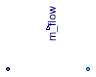### Information

This model outputs the mass flow rate flowing from `port_a` to `port_b`. The sensor is ideal, i.e., it does not influence the fluid.

Extends from Buildings.Fluid.Sensors.BaseClasses.PartialFlowSensor (Partial component to model sensors that measure flow properties), Modelica.Icons.RotationalSensor (Icon representing a round measurement device).

### Parameters

TypeNameDefaultDescription
replaceable package MediumPartialMediumMedium in the component
Nominal condition
MassFlowRatem_flow_nominal0Nominal mass flow rate, used for regularization near zero flow [kg/s]
Assumptions
BooleanallowFlowReversaltrue= false to simplify equations, assuming, but not enforcing, no flow reversal
MassFlowRatem_flow_small0For bi-directional flow, temperature is regularized in the region |m_flow| < m_flow_small (m_flow_small > 0 required) [kg/s]

### Connectors

TypeNameDescription
FluidPort_aport_aFluid connector a (positive design flow direction is from port_a to port_b)
FluidPort_bport_bFluid connector b (positive design flow direction is from port_a to port_b)
output RealOutputm_flowMass flow rate from port_a to port_b [kg/s]

### Modelica definition

model MassFlowRate "Ideal sensor for mass flow rate" extends Buildings.Fluid.Sensors.BaseClasses.PartialFlowSensor( final m_flow_nominal = 0, final m_flow_small = 0); extends Modelica.Icons.RotationalSensor; Modelica.Blocks.Interfaces.RealOutput m_flow(quantity="MassFlowRate", final unit="kg/s") "Mass flow rate from port_a to port_b"; equation m_flow = port_a.m_flow; end MassFlowRate;

##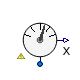Buildings.Fluid.Sensors.MassFraction

Ideal one port mass fraction sensor### Information

This model outputs the mass fraction of the fluid connected to its port. The sensor is ideal, i.e., it does not influence the fluid.

To measure mass fraction in a duct or pipe, use Buildings.Fluid.Sensors.MassFractionTwoPort rather than this sensor. Read the Buildings.Fluid.Sensors.UsersGuide prior to using this model to see about potential numerical problems if this sensor is used incorrectly in a system model.

Extends from Buildings.Fluid.Sensors.BaseClasses.PartialAbsoluteSensor (Partial component to model a sensor that measures a potential variable), Buildings.Fluid.BaseClasses.IndexMassFraction (Computes the index of a substance in the mass fraction vector Xi), Modelica.Icons.RotationalSensor (Icon representing a round measurement device).

### Parameters

TypeNameDefaultDescription
replaceable package MediumPartialMediumMedium in the sensor
StringsubstanceName"water"Name of species substance

### Connectors

TypeNameDescription
replaceable package MediumMedium in the sensor
FluidPort_aport
output RealOutputXMass fraction in port [kg/kg]

### Modelica definition

model MassFraction "Ideal one port mass fraction sensor" extends Buildings.Fluid.Sensors.BaseClasses.PartialAbsoluteSensor( redeclare replaceable package Medium = Modelica.Media.Interfaces.PartialCondensingGases); extends Buildings.Fluid.BaseClasses.IndexMassFraction(substanceName = "water"); extends Modelica.Icons.RotationalSensor; Modelica.Blocks.Interfaces.RealOutput X(min=-1e-3, max=1.001, final unit="kg/kg") "Mass fraction in port"; protected Medium.MassFraction XiVec[Medium.nXi]( final quantity=Medium.substanceNames[1:Medium.nXi]) "Mass fraction vector, needed because indexed argument for the operator inStream is not supported"; equation XiVec = inStream(port.Xi_outflow); X = if i_x > Medium.nXi then (1-sum(XiVec)) else XiVec[i_x]; end MassFraction;

##Buildings.Fluid.Sensors.MassFractionTwoPort

Ideal two port mass fraction sensor### Information

This model outputs the mass fraction of the passing fluid. The sensor is ideal, i.e. it does not influence the fluid. If the parameter `tau` is non-zero, then its output is computed using a first order differential equation. Setting `tau=0` is not recommend. See Buildings.Fluid.Sensors.UsersGuide for an explanation.

Extends from Buildings.Fluid.Sensors.BaseClasses.PartialDynamicFlowSensor (Partial component to model sensors that measure flow properties using a dynamic model), Buildings.Fluid.BaseClasses.IndexMassFraction (Computes the index of a substance in the mass fraction vector Xi), Modelica.Icons.RotationalSensor (Icon representing a round measurement device).

### Parameters

TypeNameDefaultDescription
replaceable package MediumPartialMediumMedium in the component
Timetau1Time constant at nominal flow rate (use tau=0 for steady-state sensor, but see user guide for potential problems) [s]
StringsubstanceName"water"Name of species substance
Nominal condition
MassFlowRatem_flow_nominal Nominal mass flow rate, used for regularization near zero flow [kg/s]
Initialization
InitinitTypeModelica.Blocks.Types.Init.I...Type of initialization (InitialState and InitialOutput are identical)
MassFractionX_startMedium.X_default[i_x]Initial or guess value of output (= state) [kg/kg]
Assumptions
BooleanallowFlowReversaltrue= false to simplify equations, assuming, but not enforcing, no flow reversal
MassFlowRatem_flow_small1E-4*m_flow_nominalFor bi-directional flow, temperature is regularized in the region |m_flow| < m_flow_small (m_flow_small > 0 required) [kg/s]

### Connectors

TypeNameDescription
replaceable package MediumMedium in the component
FluidPort_aport_aFluid connector a (positive design flow direction is from port_a to port_b)
FluidPort_bport_bFluid connector b (positive design flow direction is from port_a to port_b)
output RealOutputXMass fraction of the passing fluid [kg/kg]

### Modelica definition

model MassFractionTwoPort "Ideal two port mass fraction sensor" extends Buildings.Fluid.Sensors.BaseClasses.PartialDynamicFlowSensor( redeclare replaceable package Medium = Modelica.Media.Interfaces.PartialCondensingGases); extends Buildings.Fluid.BaseClasses.IndexMassFraction(substanceName = "water"); extends Modelica.Icons.RotationalSensor; parameter Medium.MassFraction X_start=Medium.X_default[i_x] "Initial or guess value of output (= state)"; Modelica.Blocks.Interfaces.RealOutput X(min=-1e-3, max=1.001, start=X_start, final unit="kg/kg") "Mass fraction of the passing fluid"; protected Medium.MassFraction XMed(start=X_start) "Mass fraction to which the sensor is exposed"; Medium.MassFraction XiVec[Medium.nXi]( final quantity=Medium.substanceNames[1:Medium.nXi]) "Mass fraction vector, needed because indexed argument for the operator inStream is not supported"; initial equation // Assign initial conditions if dynamic then if initType == Modelica.Blocks.Types.Init.SteadyState then der(X) = 0; elseif initType == Modelica.Blocks.Types.Init.InitialState or initType == Modelica.Blocks.Types.Init.InitialOutput then X = X_start; end if; end if; equation if allowFlowReversal then XiVec = Modelica.Fluid.Utilities.regStep( x=port_a.m_flow, y1=port_b.Xi_outflow, y2=port_a.Xi_outflow, x_small=m_flow_small); else XiVec = port_b.Xi_outflow; end if; XMed = if i_x > Medium.nXi then (1-sum(XiVec)) else XiVec[i_x]; // Output signal of sensor if dynamic then der(X) = (XMed-X)*k*tauInv; else X = XMed; end if; end MassFractionTwoPort;

##Buildings.Fluid.Sensors.PPM

Ideal one port trace substances sensor outputting in parts per million### Information

This model outputs the trace substance concentration in ppm contained in the fluid connected to its port. The sensor is ideal, i.e., it does not influence the fluid.

The parameter `MM` is the molar mass of the trace substance. For a list of molar masses, see Modelica.Media.IdealGases.Common.SingleGasesData and Modelica.Media.IdealGases.Common.FluidData.

To measure PPM in a duct or pipe, use Buildings.Fluid.Sensors.PPMTwoPort rather than this sensor. Read the Buildings.Fluid.Sensors.UsersGuide prior to using this model to see about potential numerical problems if this sensor is used incorrectly in a system model.

#### Assumptions

This sensor assumes that the concentration C of the medium is in mass fraction. Otherwise, the conversion to ppm will be wrong.

Extends from Buildings.Fluid.Sensors.BaseClasses.PartialAbsoluteSensor (Partial component to model a sensor that measures a potential variable), Modelica.Icons.RotationalSensor (Icon representing a round measurement device).

### Parameters

TypeNameDefaultDescription
replaceable package MediumPartialMediumMedium in the sensor
StringsubstanceName"CO2"Name of trace substance
MolarMassMMModelica.Media.IdealGases.Co...Molar mass of the trace substance [kg/mol]

### Connectors

TypeNameDescription
output RealOutputppmTrace substance in port medium in ppm

### Modelica definition

model PPM "Ideal one port trace substances sensor outputting in parts per million" extends Buildings.Fluid.Sensors.BaseClasses.PartialAbsoluteSensor( port(C_outflow(each final quantity="MassFraction", each final unit="1", each min=0, each max=1))); extends Modelica.Icons.RotationalSensor; parameter String substanceName = "CO2" "Name of trace substance"; parameter Modelica.SIunits.MolarMass MM= Modelica.Media.IdealGases.Common.SingleGasesData.CO2.MM "Molar mass of the trace substance"; Modelica.Blocks.Interfaces.RealOutput ppm(min=0) "Trace substance in port medium in ppm"; protected parameter Real s[:]= { if ( Modelica.Utilities.Strings.isEqual(string1=Medium.extraPropertiesNames[i], string2=substanceName, caseSensitive=false)) then 1 else 0 for i in 1:Medium.nC} "Vector with zero everywhere except where species is"; final parameter Modelica.SIunits.MolarMass MMBul=Medium.molarMass( Medium.setState_phX( p=Medium.p_default, h=Medium.h_default, X=Medium.X_default)) "Molar mass of bulk medium"; final parameter Real MMFraction( min=0, max=1, final unit="1", final quantity="MassFraction")=MMBul/MM "Molar mass of the medium divided by the molar mass of the trace substance"; final parameter Real coeff = MMFraction*1e6 "Conversion from mass fraction to ppm"; initial equation assert(max(s) > 0.9, "Trace substance '" + substanceName + "' is not present in medium '" + Medium.mediumName + "'.\n" + "Check sensor parameter and medium model."); equation // We obtain the species concentration with a vector multiplication // because Dymola 7.3 cannot find the derivative in the model // Buildings.Examples.VAVSystemCTControl.mo // if we set C = CVec[ind]; ppm = s*inStream(port.C_outflow)*coeff; end PPM;

##Buildings.Fluid.Sensors.PPMTwoPort

Ideal two port trace substances sensor outputting in parts per million

### Information

This model outputs the trace substance of the passing fluid in parts per million. The sensor is ideal, i.e., it does not influence the fluid. If the parameter `tau` is non-zero, then its output is computed using a first order differential equation. Setting `tau=0` is not recommend. See Buildings.Fluid.Sensors.UsersGuide for an explanation.

Extends from Buildings.Fluid.Sensors.BaseClasses.PartialDynamicFlowSensor (Partial component to model sensors that measure flow properties using a dynamic model), Modelica.Icons.RotationalSensor (Icon representing a round measurement device).

### Parameters

TypeNameDefaultDescription
replaceable package MediumPartialMediumMedium in the component
Timetau1Time constant at nominal flow rate (use tau=0 for steady-state sensor, but see user guide for potential problems) [s]
StringsubstanceName"CO2"Name of trace substance
MolarMassMMModelica.Media.IdealGases.Co...Molar mass of the trace substance [kg/mol]
Nominal condition
MassFlowRatem_flow_nominal Nominal mass flow rate, used for regularization near zero flow [kg/s]
Initialization
InitinitTypeModelica.Blocks.Types.Init.I...Type of initialization (InitialState and InitialOutput are identical)
RealC_start0Initial or guess value of output (= state)
Assumptions
BooleanallowFlowReversaltrue= false to simplify equations, assuming, but not enforcing, no flow reversal
MassFlowRatem_flow_small1E-4*m_flow_nominalFor bi-directional flow, temperature is regularized in the region |m_flow| < m_flow_small (m_flow_small > 0 required) [kg/s]

### Connectors

TypeNameDescription
output RealOutputppmTrace substance in port medium in ppm

### Modelica definition

model PPMTwoPort "Ideal two port trace substances sensor outputting in parts per million" extends Buildings.Fluid.Sensors.BaseClasses.PartialDynamicFlowSensor( port_a(C_outflow(each final quantity="MassFraction", each final unit="1", each min=0, each max=1)), port_b(C_outflow(each final quantity="MassFraction", each final unit="1", each min=0, each max=1))); extends Modelica.Icons.RotationalSensor; parameter String substanceName = "CO2" "Name of trace substance"; parameter Real C_start(min=0) = 0 "Initial or guess value of output (= state)"; parameter Modelica.SIunits.MolarMass MM= Modelica.Media.IdealGases.Common.SingleGasesData.CO2.MM "Molar mass of the trace substance"; Modelica.Blocks.Interfaces.RealOutput ppm(min=0) "Trace substance in port medium in ppm"; protected parameter Real s[:]= { if ( Modelica.Utilities.Strings.isEqual(string1=Medium.extraPropertiesNames[i], string2=substanceName, caseSensitive=false)) then 1 else 0 for i in 1:Medium.nC} "Vector with zero everywhere except where species is"; final parameter Modelica.SIunits.MolarMass MMBul=Medium.molarMass( Medium.setState_phX( p=Medium.p_default, h=Medium.h_default, X=Medium.X_default)) "Molar mass of bulk medium"; final parameter Real MMFraction(min=0, unit="1")=MMBul/MM "Molar mass of the medium divided by the molar mass of the trace substance"; final parameter Real coeff = MMFraction*1e6 "Conversion from mass fraction to ppm"; Real CMed(min=0, start=C_start, nominal=sum(Medium.C_nominal))= if allowFlowReversal then Modelica.Fluid.Utilities.regStep( x=port_a.m_flow, y1=s*port_b.C_outflow, y2=s*port_a.C_outflow, x_small=m_flow_small) else s*port_b.C_outflow "Trace substance concentration to which the sensor is exposed"; Real C(min=0,start=C_start) "Trace substance concentration of the passing fluid"; initial equation assert(max(s) > 0.9, "Trace substance '" + substanceName + "' is not present in medium '" + Medium.mediumName + "'.\n" + "Check sensor parameter and medium model."); if dynamic then if initType == Modelica.Blocks.Types.Init.SteadyState then der(C) = 0; elseif initType == Modelica.Blocks.Types.Init.InitialState or initType == Modelica.Blocks.Types.Init.InitialOutput then C = C_start; end if; end if; equation ppm = C*coeff; // Output signal of sensor if dynamic then der(C) = (CMed-C)*k*tauInv; else C = CMed; end if; end PPMTwoPort;

##Buildings.Fluid.Sensors.Pressure

Ideal pressure sensor### Information

This model outputs the absolute pressure of the fluid connected to its port. The sensor is ideal, i.e., it does not influence the fluid.

Extends from Buildings.Fluid.Sensors.BaseClasses.PartialAbsoluteSensor (Partial component to model a sensor that measures a potential variable), Modelica.Icons.RotationalSensor (Icon representing a round measurement device).

### Parameters

TypeNameDefaultDescription
replaceable package MediumPartialMediumMedium in the sensor

### Connectors

TypeNameDescription
FluidPort_aport
output RealOutputpPressure at port [Pa]

### Modelica definition

model Pressure "Ideal pressure sensor" extends Buildings.Fluid.Sensors.BaseClasses.PartialAbsoluteSensor( final warnAboutOnePortConnection = false); extends Modelica.Icons.RotationalSensor; Modelica.Blocks.Interfaces.RealOutput p(final quantity="AbsolutePressure", final unit="Pa", min=0) "Pressure at port"; equation p = port.p; end Pressure;

##Buildings.Fluid.Sensors.RelativeHumidity

Ideal one port relative humidity sensor### Information

This model outputs the relative humidity of the fluid connected to its port. The sensor is ideal, i.e. it does not influence the fluid.

Note that this sensor can only be used with media that contain the variable `phi`, which is typically the case for moist air models.

To measure relative humidity in a duct or pipe, use Buildings.Fluid.Sensors.RelativeHumidityTwoPort rather than this sensor. Read the Buildings.Fluid.Sensors.UsersGuide prior to using this model to see about potential numerical problems if this sensor is used incorrectly in a system model.

Extends from Buildings.Fluid.Sensors.BaseClasses.PartialAbsoluteSensor (Partial component to model a sensor that measures a potential variable), Modelica.Icons.RotationalSensor (Icon representing a round measurement device).

### Parameters

TypeNameDefaultDescription
replaceable package MediumPartialMediumMedium in the sensor

### Connectors

TypeNameDescription
FluidPort_aport
output RealOutputphiRelative humidity in port medium 

### Modelica definition

model RelativeHumidity "Ideal one port relative humidity sensor" extends Buildings.Fluid.Sensors.BaseClasses.PartialAbsoluteSensor; extends Modelica.Icons.RotationalSensor; Modelica.Blocks.Interfaces.RealOutput phi(final unit="1", min=0) "Relative humidity in port medium"; protected Modelica.SIunits.Temperature T "Temperature of the medium"; Medium.MassFraction Xi[Medium.nXi]( quantity=Medium.substanceNames[1:Medium.nXi]) "Mass fraction of the medium"; equation Xi = inStream(port.Xi_outflow); T=Medium.temperature_phX( p=port.p, h=inStream(port.h_outflow), X=Xi); phi = Buildings.Utilities.Psychrometrics.Functions.phi_pTX( p=port.p, T=T, X_w=Xi); end RelativeHumidity;

##Buildings.Fluid.Sensors.RelativeHumidityTwoPort

Ideal two port relative humidity sensor### Information

This model outputs the relative humidity of the fluid flowing from `port_a` to `port_b`. The sensor is ideal, i.e., it does not influence the fluid.

Note that this sensor can only be used with media that contain the variable `phi`, which is typically the case for moist air models.

If the parameter `tau` is non-zero, then its output is computed using a first order differential equation. Setting `tau=0` is not recommend. See Buildings.Fluid.Sensors.UsersGuide for an explanation.

Extends from Buildings.Fluid.Sensors.BaseClasses.PartialDynamicFlowSensor (Partial component to model sensors that measure flow properties using a dynamic model), Modelica.Icons.RotationalSensor (Icon representing a round measurement device).

### Parameters

TypeNameDefaultDescription
replaceable package MediumPartialMediumMedium in the component
Timetau1Time constant at nominal flow rate (use tau=0 for steady-state sensor, but see user guide for potential problems) [s]
Nominal condition
MassFlowRatem_flow_nominal Nominal mass flow rate, used for regularization near zero flow [kg/s]
Initialization
InitinitTypeModelica.Blocks.Types.Init.I...Type of initialization (InitialState and InitialOutput are identical)
Realphi_start0.5Initial or guess value of output (= state) 
Assumptions
BooleanallowFlowReversaltrue= false to simplify equations, assuming, but not enforcing, no flow reversal
MassFlowRatem_flow_small1E-4*m_flow_nominalFor bi-directional flow, temperature is regularized in the region |m_flow| < m_flow_small (m_flow_small > 0 required) [kg/s]

### Connectors

TypeNameDescription
FluidPort_aport_aFluid connector a (positive design flow direction is from port_a to port_b)
FluidPort_bport_bFluid connector b (positive design flow direction is from port_a to port_b)
output RealOutputphiRelative humidity of the passing fluid 

### Modelica definition

model RelativeHumidityTwoPort "Ideal two port relative humidity sensor" extends Buildings.Fluid.Sensors.BaseClasses.PartialDynamicFlowSensor; extends Modelica.Icons.RotationalSensor; Modelica.Blocks.Interfaces.RealOutput phi(final unit="1", min=0, start=phi_start) "Relative humidity of the passing fluid"; parameter Real phi_start(final unit="1", min=0, max=1)=0.5 "Initial or guess value of output (= state)"; protected Real phiMed(final unit="1", min=0, start=phi_start) "Relative humidity to which the sensor is exposed to"; protected Modelica.SIunits.Temperature T_a "Temperature of the medium flowing from port_a to port_b"; Medium.MassFraction Xi_a[Medium.nXi]( quantity=Medium.substanceNames[1:Medium.nXi]) "Mass fraction of the medium flowing from port_a to port_b"; Real phi_a(final unit="1") "Relative humidity of the medium flowing from port_a to port_b"; Modelica.SIunits.Temperature T_b "Temperature of the medium flowing from port_b to port_a"; Medium.MassFraction Xi_b[Medium.nXi]( quantity=Medium.substanceNames[1:Medium.nXi]) "Mass fraction of the medium flowing from port_b to port_a"; Real phi_b(final unit="1") "Relative humidity of the medium flowing from port_b to port_a"; initial equation if dynamic then if initType == Modelica.Blocks.Types.Init.SteadyState then der(phi) = 0; elseif initType == Modelica.Blocks.Types.Init.InitialState or initType == Modelica.Blocks.Types.Init.InitialOutput then phi = phi_start; end if; end if; equation // Since the sensor does not affect the medium, we can use // port_b.Xi_outflow = inStream(port_a.Xi_outflow). Xi_a = port_b.Xi_outflow; T_a=Medium.temperature_phX( p=port_a.p, h=port_b.h_outflow, X=Xi_a); phi_a = Buildings.Utilities.Psychrometrics.Functions.phi_pTX( p=port_a.p, T=T_a, X_w=Xi_a); if allowFlowReversal then phi_b = Buildings.Utilities.Psychrometrics.Functions.phi_pTX( p=port_b.p, T=T_b, X_w=Xi_b); T_b=Medium.temperature_phX( p=port_b.p, h=port_a.h_outflow, X=Xi_b); Xi_b = port_a.Xi_outflow; phiMed = Modelica.Fluid.Utilities.regStep( x=port_a.m_flow, y1=phi_a, y2=phi_b, x_small=m_flow_small); else phi_b = 0; T_b = 273.15; Xi_b = zeros(Medium.nXi); phiMed = phi_a; end if; // Output signal of sensor if dynamic then der(phi) = (phiMed-phi)*k*tauInv; else phi = phiMed; end if; end RelativeHumidityTwoPort;

##Buildings.Fluid.Sensors.RelativePressure

Ideal relative pressure sensor### Information

The relative pressure `port_a.p - port_b.p` is determined between the two ports of this component and is provided as output signal. The sensor should be connected in parallel with other equipment, no flow through the sensor is allowed.

Extends from Modelica.Icons.TranslationalSensor (Icon representing a linear measurement device).

### Parameters

TypeNameDefaultDescription
replaceable package MediumModelica.Media.Interfaces.Pa...Medium in the sensor

### Connectors

TypeNameDescription
replaceable package MediumMedium in the sensor
FluidPort_aport_aFluid connector of stream a
FluidPort_bport_bFluid connector of stream b
output RealOutputp_relRelative pressure of port_a minus port_b [Pa]

### Modelica definition

model RelativePressure "Ideal relative pressure sensor" extends Modelica.Icons.TranslationalSensor; replaceable package Medium = Modelica.Media.Interfaces.PartialMedium "Medium in the sensor"; Modelica.Fluid.Interfaces.FluidPort_a port_a(m_flow(min=0), p(start=Medium.p_default), redeclare package Medium = Medium) "Fluid connector of stream a"; Modelica.Fluid.Interfaces.FluidPort_b port_b(m_flow(min=0), p(start=Medium.p_default), redeclare package Medium = Medium) "Fluid connector of stream b"; Modelica.Blocks.Interfaces.RealOutput p_rel(final quantity="PressureDifference", final unit="Pa", displayUnit="Pa") "Relative pressure of port_a minus port_b"; equation // Zero flow equations for connectors port_a.m_flow = 0; port_b.m_flow = 0; // No contribution of specific quantities port_a.h_outflow = 0; port_b.h_outflow = 0; port_a.Xi_outflow = zeros(Medium.nXi); port_b.Xi_outflow = zeros(Medium.nXi); port_a.C_outflow = zeros(Medium.nC); port_b.C_outflow = zeros(Medium.nC); // Relative pressure p_rel = port_a.p - port_b.p; end RelativePressure;

##Buildings.Fluid.Sensors.RelativeTemperature

Ideal relative temperature sensor### Information

The relative temperature `T(port_a) - T(port_b)` is determined between the two ports of this component and is provided as output signal. The sensor should be connected in parallel with other equipment. There is no flow through the sensor.

Note that this sensor should only be connected to fluid volumes, such as Buildings.Fluid.MixingVolumes.MixingVolume. Otherwise, numerical problems may occur if one of the mass flow rates are close to zero. See Buildings.Fluid.Sensors.UsersGuide for an explanation.

Extends from Modelica.Icons.TranslationalSensor (Icon representing a linear measurement device).

### Parameters

TypeNameDefaultDescription
replaceable package MediumModelica.Media.Interfaces.Pa...Medium in the sensor

### Connectors

TypeNameDescription
replaceable package MediumMedium in the sensor
FluidPort_aport_aFluid connector of stream a
FluidPort_bport_bFluid connector of stream b
output RealOutputT_relTemperature difference of port_a minus port_b [K]

### Modelica definition

model RelativeTemperature "Ideal relative temperature sensor" extends Modelica.Icons.TranslationalSensor; replaceable package Medium = Modelica.Media.Interfaces.PartialMedium "Medium in the sensor"; Modelica.Fluid.Interfaces.FluidPort_a port_a(m_flow(min=0), p(start=Medium.p_default), redeclare package Medium = Medium) "Fluid connector of stream a"; Modelica.Fluid.Interfaces.FluidPort_b port_b(m_flow(min=0), p(start=Medium.p_default), redeclare package Medium = Medium) "Fluid connector of stream b"; Modelica.Blocks.Interfaces.RealOutput T_rel(final unit = "K", displayUnit = "K") "Temperature difference of port_a minus port_b"; equation // Zero flow equations for connectors port_a.m_flow = 0; port_b.m_flow = 0; // No contribution of specific quantities port_a.h_outflow = 0; port_b.h_outflow = 0; port_a.Xi_outflow = zeros(Medium.nXi); port_b.Xi_outflow = zeros(Medium.nXi); port_a.C_outflow = zeros(Medium.nC); port_b.C_outflow = zeros(Medium.nC); // Relative temperature T_rel = Medium.temperature(state=Medium.setState_phX( p=port_a.p, h=inStream(port_a.h_outflow), X=inStream(port_a.Xi_outflow))) - Medium.temperature(state=Medium.setState_phX( p=port_b.p, h=inStream(port_b.h_outflow), X=inStream(port_b.Xi_outflow))); end RelativeTemperature;

##Buildings.Fluid.Sensors.SensibleEnthalpyFlowRate

Ideal enthalphy flow rate sensor that outputs the sensible enthalpy flow rate only### Information

This model outputs the sensible enthalphy flow rate of the medium in the flow between its fluid ports. In particular, if the total enthalpy flow rate is

tot = Ḣsen + Ḣlat,

where sen = ṁ (1-Xw) cp,air, then this sensor outputs Ḣ = Ḣsen.

If the parameter `tau` is non-zero, then the measured specific sensible enthalpy hout that is used to compute the sensible enthalpy flow rate sen = ṁ hout is computed using a first order differential equation. See Buildings.Fluid.Sensors.UsersGuide for an explanation.

For a sensor that measures tot, use Buildings.Fluid.Sensors.EnthalpyFlowRate.
For a sensor that measures lat, use Buildings.Fluid.Sensors.LatentEnthalpyFlowRate.

The sensor is ideal, i.e., it does not influence the fluid. The sensor can only be used with medium models that implement the function `enthalpyOfNonCondensingGas(T)`.

Extends from Buildings.Fluid.Sensors.BaseClasses.PartialDynamicFlowSensor (Partial component to model sensors that measure flow properties using a dynamic model), Buildings.Fluid.BaseClasses.IndexMassFraction (Computes the index of a substance in the mass fraction vector Xi), Modelica.Icons.RotationalSensor (Icon representing a round measurement device).

### Parameters

TypeNameDefaultDescription
replaceable package MediumPartialMediumMedium in the component
Timetau0Time constant at nominal flow rate (use tau=0 for steady-state sensor, but see user guide for potential problems) [s]
StringsubstanceName"water"Name of species substance
Nominal condition
MassFlowRatem_flow_nominal Nominal mass flow rate, used for regularization near zero flow [kg/s]
Initialization
InitinitTypeModelica.Blocks.Types.Init.I...Type of initialization (InitialState and InitialOutput are identical)
SpecificEnthalpyh_out_startMedium.enthalpyOfNonCondensi...Initial or guess value of measured specific sensible enthalpy [J/kg]
Assumptions
BooleanallowFlowReversaltrue= false to simplify equations, assuming, but not enforcing, no flow reversal
MassFlowRatem_flow_small1E-4*m_flow_nominalFor bi-directional flow, temperature is regularized in the region |m_flow| < m_flow_small (m_flow_small > 0 required) [kg/s]

### Connectors

TypeNameDescription
replaceable package MediumMedium in the component
FluidPort_aport_aFluid connector a (positive design flow direction is from port_a to port_b)
FluidPort_bport_bFluid connector b (positive design flow direction is from port_a to port_b)
output RealOutputH_flowSensible enthalpy flow rate, positive if from port_a to port_b [W]

### Modelica definition

model SensibleEnthalpyFlowRate "Ideal enthalphy flow rate sensor that outputs the sensible enthalpy flow rate only" extends Buildings.Fluid.Sensors.BaseClasses.PartialDynamicFlowSensor( redeclare replaceable package Medium = Modelica.Media.Interfaces.PartialCondensingGases, tau=0); extends Buildings.Fluid.BaseClasses.IndexMassFraction(final substanceName="water"); extends Modelica.Icons.RotationalSensor; Modelica.Blocks.Interfaces.RealOutput H_flow(final unit="W") "Sensible enthalpy flow rate, positive if from port_a to port_b"; parameter Modelica.SIunits.SpecificEnthalpy h_out_start= Medium.enthalpyOfNonCondensingGas(T=Medium.T_default) "Initial or guess value of measured specific sensible enthalpy"; protected Modelica.SIunits.SpecificEnthalpy hMed_out(start=h_out_start) "Medium sensible enthalpy to which the sensor is exposed"; Modelica.SIunits.SpecificEnthalpy h_out(start=h_out_start) "Medium sensible enthalpy that is used to compute the enthalpy flow rate"; Medium.MassFraction XiActual[Medium.nXi] "Medium mass fraction to which sensor is exposed to"; Medium.SpecificEnthalpy hActual "Medium enthalpy to which sensor is exposed to"; initial equation // Compute initial state if dynamic then if initType == Modelica.Blocks.Types.Init.SteadyState then der(h_out) = 0; elseif initType == Modelica.Blocks.Types.Init.InitialState or initType == Modelica.Blocks.Types.Init.InitialOutput then h_out = h_out_start; end if; end if; equation if allowFlowReversal then XiActual = Modelica.Fluid.Utilities.regStep( x=port_a.m_flow, y1=port_b.Xi_outflow, y2=port_a.Xi_outflow, x_small=m_flow_small); hActual = Modelica.Fluid.Utilities.regStep( x=port_a.m_flow, y1=port_b.h_outflow, y2=port_a.h_outflow, x_small=m_flow_small); else XiActual = port_b.Xi_outflow; hActual = port_b.h_outflow; end if; // Specific enthalpy measured by sensor hMed_out = (1-XiActual[i_x]) * Medium.enthalpyOfNonCondensingGas( T=Medium.temperature(state=Medium.setState_phX(p=port_a.p, h=hActual, X=XiActual))); if dynamic then der(h_out) = (hMed_out-h_out)*k*tauInv; else h_out = hMed_out; end if; // Sensor output signal H_flow = port_a.m_flow * h_out; end SensibleEnthalpyFlowRate;

##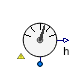Buildings.Fluid.Sensors.SpecificEnthalpy

Ideal one port specific enthalpy sensor### Information

This model outputs the specific enthalpy of the fluid connected to its port. The sensor is ideal, i.e. it does not influence the fluid.

To measure specific enthalpy in a duct or pipe, use Buildings.Fluid.Sensors.SpecificEnthalpyTwoPort rather than this sensor. Read the Buildings.Fluid.Sensors.UsersGuide prior to using this model to see about potential numerical problems if this sensor is used incorrectly in a system model.

Extends from Buildings.Fluid.Sensors.BaseClasses.PartialAbsoluteSensor (Partial component to model a sensor that measures a potential variable), Modelica.Icons.RotationalSensor (Icon representing a round measurement device).

### Parameters

TypeNameDefaultDescription
replaceable package MediumPartialMediumMedium in the sensor

### Connectors

TypeNameDescription
FluidPort_aport
output RealOutputh_outSpecific enthalpy in port medium [J/kg]

### Modelica definition

model SpecificEnthalpy "Ideal one port specific enthalpy sensor" extends Buildings.Fluid.Sensors.BaseClasses.PartialAbsoluteSensor; extends Modelica.Icons.RotationalSensor; Modelica.Blocks.Interfaces.RealOutput h_out(final quantity="SpecificEnergy", final unit="J/kg") "Specific enthalpy in port medium"; equation h_out = inStream(port.h_outflow); end SpecificEnthalpy;

##Buildings.Fluid.Sensors.SpecificEnthalpyTwoPort

Ideal two port sensor for the specific enthalpy### Information

This model outputs the specific enthalpy of a passing fluid. The sensor is ideal, i.e. it does not influence the fluid. If the parameter `tau` is non-zero, then its output is computed using a first order differential equation. Setting `tau=0` is not recommend. See Buildings.Fluid.Sensors.UsersGuide for an explanation.

Extends from Buildings.Fluid.Sensors.BaseClasses.PartialDynamicFlowSensor (Partial component to model sensors that measure flow properties using a dynamic model), Modelica.Icons.RotationalSensor (Icon representing a round measurement device).

### Parameters

TypeNameDefaultDescription
replaceable package MediumPartialMediumMedium in the component
Timetau1Time constant at nominal flow rate (use tau=0 for steady-state sensor, but see user guide for potential problems) [s]
Nominal condition
MassFlowRatem_flow_nominal Nominal mass flow rate, used for regularization near zero flow [kg/s]
Initialization
InitinitTypeModelica.Blocks.Types.Init.I...Type of initialization (InitialState and InitialOutput are identical)
SpecificEnthalpyh_out_startMedium.specificEnthalpy_pTX(...Initial or guess value of output (= state) [J/kg]
Assumptions
BooleanallowFlowReversaltrue= false to simplify equations, assuming, but not enforcing, no flow reversal
MassFlowRatem_flow_small1E-4*m_flow_nominalFor bi-directional flow, temperature is regularized in the region |m_flow| < m_flow_small (m_flow_small > 0 required) [kg/s]

### Connectors

TypeNameDescription
FluidPort_aport_aFluid connector a (positive design flow direction is from port_a to port_b)
FluidPort_bport_bFluid connector b (positive design flow direction is from port_a to port_b)
output RealOutputh_outSpecific enthalpy of the passing fluid [J/kg]

### Modelica definition

model SpecificEnthalpyTwoPort "Ideal two port sensor for the specific enthalpy" extends Buildings.Fluid.Sensors.BaseClasses.PartialDynamicFlowSensor; extends Modelica.Icons.RotationalSensor; parameter Modelica.SIunits.SpecificEnthalpy h_out_start= Medium.specificEnthalpy_pTX(p=Medium.p_default, T=Medium.T_default, X=Medium.X_default) "Initial or guess value of output (= state)"; Modelica.Blocks.Interfaces.RealOutput h_out(final quantity="SpecificEnergy", final unit="J/kg", start=h_out_start) "Specific enthalpy of the passing fluid"; protected Modelica.SIunits.SpecificEnthalpy hMed_out(start=h_out_start) "Medium enthalpy to which the sensor is exposed"; initial equation if dynamic then if initType == Modelica.Blocks.Types.Init.SteadyState then der(h_out) = 0; elseif initType == Modelica.Blocks.Types.Init.InitialState or initType == Modelica.Blocks.Types.Init.InitialOutput then h_out = h_out_start; end if; end if; equation if allowFlowReversal then hMed_out = Modelica.Fluid.Utilities.regStep( x=port_a.m_flow, y1=port_b.h_outflow, y2=port_a.h_outflow, x_small=m_flow_small); else hMed_out = port_b.h_outflow; end if; // Output signal of sensor if dynamic then der(h_out) = (hMed_out-h_out)*k*tauInv; else h_out = hMed_out; end if; end SpecificEnthalpyTwoPort;

##Buildings.Fluid.Sensors.SpecificEntropy

Ideal one port specific entropy sensor### Information

This model outputs the specific entropy of the fluid connected to its port. The sensor is ideal, i.e., it does not influence the fluid.

To measure specific entropy in a duct or pipe, use Buildings.Fluid.Sensors.SpecificEntropyTwoPort rather than this sensor. Read the Buildings.Fluid.Sensors.UsersGuide prior to using this model to see about potential numerical problems if this sensor is used incorrectly in a system model.

Extends from Buildings.Fluid.Sensors.BaseClasses.PartialAbsoluteSensor (Partial component to model a sensor that measures a potential variable), Modelica.Icons.RotationalSensor (Icon representing a round measurement device).

### Parameters

TypeNameDefaultDescription
replaceable package MediumPartialMediumMedium in the sensor

### Connectors

TypeNameDescription
FluidPort_aport
output RealOutputsSpecific entropy in port medium [J/(kg.K)]

### Modelica definition

model SpecificEntropy "Ideal one port specific entropy sensor" extends Buildings.Fluid.Sensors.BaseClasses.PartialAbsoluteSensor; extends Modelica.Icons.RotationalSensor; Modelica.Blocks.Interfaces.RealOutput s(final quantity="SpecificEntropy", final unit="J/(kg.K)") "Specific entropy in port medium"; equation s = Medium.specificEntropy(state=Medium.setState_phX( p=port.p, h=inStream(port.h_outflow), X=inStream(port.Xi_outflow))); end SpecificEntropy;

##Buildings.Fluid.Sensors.SpecificEntropyTwoPort

Ideal two port sensor for the specific entropy### Information

This model outputs the specific entropy of the passing fluid. The sensor is ideal, i.e., it does not influence the fluid. If the parameter `tau` is non-zero, then its output is computed using a first order differential equation. Setting `tau=0` is not recommend. See Buildings.Fluid.Sensors.UsersGuide for an explanation.

Extends from Buildings.Fluid.Sensors.BaseClasses.PartialDynamicFlowSensor (Partial component to model sensors that measure flow properties using a dynamic model), Modelica.Icons.RotationalSensor (Icon representing a round measurement device).

### Parameters

TypeNameDefaultDescription
replaceable package MediumPartialMediumMedium in the component
Timetau1Time constant at nominal flow rate (use tau=0 for steady-state sensor, but see user guide for potential problems) [s]
Nominal condition
MassFlowRatem_flow_nominal Nominal mass flow rate, used for regularization near zero flow [kg/s]
Initialization
InitinitTypeModelica.Blocks.Types.Init.I...Type of initialization (InitialState and InitialOutput are identical)
SpecificEntropys_startMedium.specificEntropy_pTX(p...Initial or guess value of output (= state) [J/(kg.K)]
Assumptions
BooleanallowFlowReversaltrue= false to simplify equations, assuming, but not enforcing, no flow reversal
MassFlowRatem_flow_small1E-4*m_flow_nominalFor bi-directional flow, temperature is regularized in the region |m_flow| < m_flow_small (m_flow_small > 0 required) [kg/s]

### Connectors

TypeNameDescription
FluidPort_aport_aFluid connector a (positive design flow direction is from port_a to port_b)
FluidPort_bport_bFluid connector b (positive design flow direction is from port_a to port_b)
output RealOutputsSpecific entropy of the passing fluid [J/(kg.K)]

### Modelica definition

model SpecificEntropyTwoPort "Ideal two port sensor for the specific entropy" extends Buildings.Fluid.Sensors.BaseClasses.PartialDynamicFlowSensor; extends Modelica.Icons.RotationalSensor; parameter Modelica.SIunits.SpecificEntropy s_start= Medium.specificEntropy_pTX(p=Medium.p_default, T=Medium.T_default, X=Medium.X_default) "Initial or guess value of output (= state)"; Modelica.Blocks.Interfaces.RealOutput s(final quantity="SpecificEntropy", final unit="J/(kg.K)", start=s_start) "Specific entropy of the passing fluid"; protected Modelica.SIunits.SpecificEntropy sMed(start=s_start) "Medium entropy to which the sensor is exposed"; Medium.SpecificEntropy s_a_inflow "Specific entropy of inflowing fluid at port_a"; Medium.SpecificEntropy s_b_inflow "Specific entropy of inflowing fluid at port_b, or s_a_inflow if uni-directional flow"; initial equation if dynamic then if initType == Modelica.Blocks.Types.Init.SteadyState then der(s) = 0; elseif initType == Modelica.Blocks.Types.Init.InitialState or initType == Modelica.Blocks.Types.Init.InitialOutput then s = s_start; end if; end if; equation if allowFlowReversal then s_a_inflow = Medium.specificEntropy(state= Medium.setState_phX(p=port_b.p, h=port_b.h_outflow, X=port_b.Xi_outflow)); s_b_inflow = Medium.specificEntropy(state= Medium.setState_phX(p=port_a.p, h=port_a.h_outflow, X=port_a.Xi_outflow)); sMed = Modelica.Fluid.Utilities.regStep( x=port_a.m_flow, y1=s_a_inflow, y2=s_b_inflow, x_small=m_flow_small); else sMed = Medium.specificEntropy(state= Medium.setState_phX(p=port_b.p, h=port_b.h_outflow, X=port_b.Xi_outflow)); s_a_inflow = sMed; s_b_inflow = sMed; end if; // Output signal of sensor if dynamic then der(s) = (sMed-s)*k*tauInv; else s = sMed; end if; end SpecificEntropyTwoPort;

##Buildings.Fluid.Sensors.Temperature

Ideal one port temperature sensor### Information

This model outputs the temperature of the fluid connected to its port. The sensor is ideal, i.e., it does not influence the fluid.

To measure temperature in a duct or pipe, use Buildings.Fluid.Sensors.TemperatureTwoPort rather than this sensor. Read the Buildings.Fluid.Sensors.UsersGuide prior to using this model to see about potential numerical problems if this sensor is used incorrectly in a system model.

Extends from Buildings.Fluid.Sensors.BaseClasses.PartialAbsoluteSensor (Partial component to model a sensor that measures a potential variable).

### Parameters

TypeNameDefaultDescription
replaceable package MediumPartialMediumMedium in the sensor

### Connectors

TypeNameDescription
FluidPort_aport
output RealOutputTTemperature in port medium [K]

### Modelica definition

model Temperature "Ideal one port temperature sensor" extends Buildings.Fluid.Sensors.BaseClasses.PartialAbsoluteSensor; Modelica.Blocks.Interfaces.RealOutput T(final quantity="ThermodynamicTemperature", final unit = "K", min=0, displayUnit = "degC") "Temperature in port medium"; equation T = Medium.temperature(state=Medium.setState_phX( p=port.p, h=inStream(port.h_outflow), X=inStream(port.Xi_outflow))); end Temperature;

##Buildings.Fluid.Sensors.TemperatureTwoPort

Ideal two port temperature sensor### Information

This model outputs the temperature of the medium in the flow between its fluid ports. The sensor does not influence the fluid.

#### Typical use and important parameters

If the parameter `tau` is non-zero, then its output T converges to the temperature of the incoming fluid using a first order differential equation. Setting `tau=0` is not recommend. See Buildings.Fluid.Sensors.UsersGuide for an explanation.

If `transferHeat = true`, then heat transfer with the ambient is approximated and T converges towards the fixed ambient temperature TAmb using a first order approximation with a time constant of `tauHeaTra` when the flow rate is small. Note that no energy is exchanged with the fluid as the sensor does not influence the fluid temperature.

Setting `transferHeat = true` is useful, for example, if the sensor is used to measure the fluid temperature in a system with on/off control on the mass flow rate. If `transferHeat` were `false`, then the sensor output T would remain constant if the mass flow rate is set to zero, and hence the controller may never switch the mass flow rate on again.

In general, applications in which the sensor output is not used to switch the mass flow rate on should set `transferHeat=false`.

Extends from Buildings.Fluid.Sensors.BaseClasses.PartialDynamicFlowSensor (Partial component to model sensors that measure flow properties using a dynamic model).

### Parameters

TypeNameDefaultDescription
replaceable package MediumPartialMediumMedium in the component
Timetau1Time constant at nominal flow rate (use tau=0 for steady-state sensor, but see user guide for potential problems) [s]
Nominal condition
MassFlowRatem_flow_nominal Nominal mass flow rate, used for regularization near zero flow [kg/s]
Initialization
InitinitTypeModelica.Blocks.Types.Init.I...Type of initialization (InitialState and InitialOutput are identical)
TemperatureT_startMedium.T_defaultInitial or guess value of output (= state) [K]
Heat transfer
BooleantransferHeatfalseif true, temperature T converges towards TAmb when no flow
TemperatureTAmbMedium.T_defaultFixed ambient temperature for heat transfer [K]
TimetauHeaTra1200Time constant for heat transfer, default 20 minutes [s]
Assumptions
BooleanallowFlowReversaltrue= false to simplify equations, assuming, but not enforcing, no flow reversal
MassFlowRatem_flow_small1E-4*m_flow_nominalFor bi-directional flow, temperature is regularized in the region |m_flow| < m_flow_small (m_flow_small > 0 required) [kg/s]

### Connectors

TypeNameDescription
FluidPort_aport_aFluid connector a (positive design flow direction is from port_a to port_b)
FluidPort_bport_bFluid connector b (positive design flow direction is from port_a to port_b)
output RealOutputTTemperature of the passing fluid [K]

### Modelica definition

model TemperatureTwoPort "Ideal two port temperature sensor" extends Buildings.Fluid.Sensors.BaseClasses.PartialDynamicFlowSensor; Modelica.Blocks.Interfaces.RealOutput T(final quantity="ThermodynamicTemperature", final unit="K", displayUnit = "degC", min = 0, start=T_start) "Temperature of the passing fluid"; parameter Modelica.SIunits.Temperature T_start=Medium.T_default "Initial or guess value of output (= state)"; parameter Boolean transferHeat = false "if true, temperature T converges towards TAmb when no flow"; parameter Modelica.SIunits.Temperature TAmb=Medium.T_default "Fixed ambient temperature for heat transfer"; parameter Modelica.SIunits.Time tauHeaTra(min=1)=1200 "Time constant for heat transfer, default 20 minutes"; protected parameter Real tauHeaTraInv(final unit = "1/s")= if tauHeaTra<1E-10 then 0 else 1/tauHeaTra "Dummy parameter to avoid division by tauHeaTra"; parameter Real ratTau = if dynamic then tauHeaTra/tau else 1 "Ratio of tau"; Medium.Temperature TMed(start=T_start) "Medium temperature to which the sensor is exposed"; Medium.Temperature T_a_inflow "Temperature of inflowing fluid at port_a"; Medium.Temperature T_b_inflow "Temperature of inflowing fluid at port_b, or T_a_inflow if uni-directional flow"; initial equation if dynamic then if initType == Modelica.Blocks.Types.Init.SteadyState then der(T) = 0; elseif initType == Modelica.Blocks.Types.Init.InitialState or initType == Modelica.Blocks.Types.Init.InitialOutput then T = T_start; end if; end if; equation if allowFlowReversal then T_a_inflow = Medium.temperature(state= Medium.setState_phX(p=port_b.p, h=port_b.h_outflow, X=port_b.Xi_outflow)); T_b_inflow = Medium.temperature(state= Medium.setState_phX(p=port_a.p, h=port_a.h_outflow, X=port_a.Xi_outflow)); TMed = Modelica.Fluid.Utilities.regStep( x=port_a.m_flow, y1=T_a_inflow, y2=T_b_inflow, x_small=m_flow_small); else TMed = Medium.temperature(state= Medium.setState_phX(p=port_b.p, h=port_b.h_outflow, X=port_b.Xi_outflow)); T_a_inflow = TMed; T_b_inflow = TMed; end if; // Output signal of sensor if dynamic then if transferHeat then der(T) = (TMed-T)*k*tauInv + (TAmb-T)*tauHeaTraInv/(ratTau*k+1); else der(T) = (TMed-T)*k*tauInv; end if; else T = TMed; end if; end TemperatureTwoPort;

##Buildings.Fluid.Sensors.TemperatureWetBulbTwoPort

Ideal wet bulb temperature sensor### Information

This sensor outputs the wet bulb temperature of the medium in the flow between its fluid ports. The sensor is ideal, i.e., it does not influence the fluid. If the parameter `tau` is non-zero, then its output is computed using a first order differential equation. Setting `tau=0` is not recommend. See Buildings.Fluid.Sensors.UsersGuide for an explanation.

Extends from Buildings.Fluid.Sensors.BaseClasses.PartialDynamicFlowSensor (Partial component to model sensors that measure flow properties using a dynamic model).

### Parameters

TypeNameDefaultDescription
replaceable package MediumPartialMediumMedium in the component
Timetau1Time constant at nominal flow rate (use tau=0 for steady-state sensor, but see user guide for potential problems) [s]
Nominal condition
MassFlowRatem_flow_nominal Nominal mass flow rate, used for regularization near zero flow [kg/s]
Initialization
InitinitTypeModelica.Blocks.Types.Init.I...Type of initialization (InitialState and InitialOutput are identical)
TemperatureTWetBul_startMedium.T_defaultInitial or guess value of wet bulb temperature (used to compute initial output signal)) [K]
Assumptions
BooleanallowFlowReversaltrue= false to simplify equations, assuming, but not enforcing, no flow reversal
MassFlowRatem_flow_small1E-4*m_flow_nominalFor bi-directional flow, temperature is regularized in the region |m_flow| < m_flow_small (m_flow_small > 0 required) [kg/s]

### Connectors

TypeNameDescription
FluidPort_aport_aFluid connector a (positive design flow direction is from port_a to port_b)
FluidPort_bport_bFluid connector b (positive design flow direction is from port_a to port_b)
output RealOutputTWet bulb temperature in port medium [K]

### Modelica definition

model TemperatureWetBulbTwoPort "Ideal wet bulb temperature sensor" extends Buildings.Fluid.Sensors.BaseClasses.PartialDynamicFlowSensor; Modelica.Blocks.Interfaces.RealOutput T( start=TWetBul_start, final quantity="ThermodynamicTemperature", final unit="K", displayUnit = "degC") "Wet bulb temperature in port medium"; parameter Modelica.SIunits.Temperature TWetBul_start = Medium.T_default "Initial or guess value of wet bulb temperature (used to compute initial output signal))"; protected Medium.Temperature TMedWetBul(start=TWetBul_start) "Medium wet bulb temperature to which the sensor is exposed"; Buildings.Utilities.Psychrometrics.TWetBul_TDryBulXi wetBulMod( redeclare package Medium = Medium, TWetBul(start=TWetBul_start)) "Block for wet bulb temperature"; Modelica.SIunits.SpecificEnthalpy h "Specific enthalpy"; Medium.MassFraction Xi[Medium.nXi] "Species vector, needed because indexed argument for the operator inStream is not supported"; initial equation // Initialization of wet bulb temperature if dynamic then if initType == Modelica.Blocks.Types.Init.SteadyState then der(T) = 0; elseif initType == Modelica.Blocks.Types.Init.InitialState or initType == Modelica.Blocks.Types.Init.InitialOutput then T = TWetBul_start; end if; end if; equation if allowFlowReversal then h = Modelica.Fluid.Utilities.regStep( x=port_a.m_flow, y1=port_b.h_outflow, y2=port_a.h_outflow, x_small=m_flow_small); Xi = Modelica.Fluid.Utilities.regStep( x=port_a.m_flow, y1=port_b.Xi_outflow, y2=port_a.Xi_outflow, x_small=m_flow_small); else h = port_b.h_outflow; Xi = port_b.Xi_outflow; end if; // Compute wet bulb temperature wetBulMod.TDryBul = Medium.temperature_phX(p=port_a.p, h=h, X=cat(1,Xi,{1-sum(Xi)})); wetBulMod.Xi = Xi; wetBulMod.p = port_a.p; TMedWetBul = wetBulMod.TWetBul; // Output signal of sensor if dynamic then der(T) = (TMedWetBul-T)*k*tauInv; else T = TMedWetBul; end if; end TemperatureWetBulbTwoPort;

##Buildings.Fluid.Sensors.TraceSubstances

Ideal one port trace substances sensor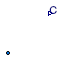### Information

This model outputs the trace substances contained in the fluid connected to its port. The sensor is ideal, i.e., it does not influence the fluid.

To measure trace substances in a duct or pipe, use Buildings.Fluid.Sensors.TraceSubstancesTwoPort rather than this sensor. Read the Buildings.Fluid.Sensors.UsersGuide prior to using this model to see about potential numerical problems if this sensor is used incorrectly in a system model.

Extends from Buildings.Fluid.Sensors.BaseClasses.PartialAbsoluteSensor (Partial component to model a sensor that measures a potential variable), Modelica.Icons.RotationalSensor (Icon representing a round measurement device).

### Parameters

TypeNameDefaultDescription
replaceable package MediumPartialMediumMedium in the sensor
StringsubstanceName"CO2"Name of trace substance

### Connectors

TypeNameDescription
FluidPort_aport
output RealOutputCTrace substance in port medium

### Modelica definition

model TraceSubstances "Ideal one port trace substances sensor" extends Buildings.Fluid.Sensors.BaseClasses.PartialAbsoluteSensor; extends Modelica.Icons.RotationalSensor; parameter String substanceName = "CO2" "Name of trace substance"; Modelica.Blocks.Interfaces.RealOutput C(min=0) "Trace substance in port medium"; protected parameter Real s[:]= { if ( Modelica.Utilities.Strings.isEqual(string1=Medium.extraPropertiesNames[i], string2=substanceName, caseSensitive=false)) then 1 else 0 for i in 1:Medium.nC} "Vector with zero everywhere except where species is"; initial equation assert(max(s) > 0.9, "Trace substance '" + substanceName + "' is not present in medium '" + Medium.mediumName + "'.\n" + "Check sensor parameter and medium model."); equation // We obtain the species concentration with a vector multiplication // because Dymola 7.3 cannot find the derivative in the model // Buildings.Examples.VAVSystemCTControl.mo // if we set C = CVec[ind]; C = s*inStream(port.C_outflow); end TraceSubstances;

##Buildings.Fluid.Sensors.TraceSubstancesTwoPort

Ideal two port sensor for trace substance### Information

This model outputs the trace substance of the passing fluid. The sensor is ideal, i.e., it does not influence the fluid. If the parameter `tau` is non-zero, then its output is computed using a first order differential equation. Setting `tau=0` is not recommend. See Buildings.Fluid.Sensors.UsersGuide for an explanation.

Extends from Buildings.Fluid.Sensors.BaseClasses.PartialDynamicFlowSensor (Partial component to model sensors that measure flow properties using a dynamic model), Modelica.Icons.RotationalSensor (Icon representing a round measurement device).

### Parameters

TypeNameDefaultDescription
replaceable package MediumPartialMediumMedium in the component
Timetau1Time constant at nominal flow rate (use tau=0 for steady-state sensor, but see user guide for potential problems) [s]
StringsubstanceName"CO2"Name of trace substance
Nominal condition
MassFlowRatem_flow_nominal Nominal mass flow rate, used for regularization near zero flow [kg/s]
Initialization
InitinitTypeModelica.Blocks.Types.Init.I...Type of initialization (InitialState and InitialOutput are identical)
RealC_start0Initial or guess value of output (= state)
Assumptions
BooleanallowFlowReversaltrue= false to simplify equations, assuming, but not enforcing, no flow reversal
MassFlowRatem_flow_small1E-4*m_flow_nominalFor bi-directional flow, temperature is regularized in the region |m_flow| < m_flow_small (m_flow_small > 0 required) [kg/s]

### Connectors

TypeNameDescription
FluidPort_aport_aFluid connector a (positive design flow direction is from port_a to port_b)
FluidPort_bport_bFluid connector b (positive design flow direction is from port_a to port_b)
output RealOutputCTrace substance of the passing fluid

### Modelica definition

model TraceSubstancesTwoPort "Ideal two port sensor for trace substance" extends Buildings.Fluid.Sensors.BaseClasses.PartialDynamicFlowSensor; extends Modelica.Icons.RotationalSensor; Modelica.Blocks.Interfaces.RealOutput C(min=0, start=C_start) "Trace substance of the passing fluid"; parameter String substanceName = "CO2" "Name of trace substance"; parameter Real C_start(min=0) = 0 "Initial or guess value of output (= state)"; protected constant Real sumC_nominal = sum(Medium.C_nominal) "Sum of Medium.C_nominal"; Real CMed(min=0, start=C_start, nominal= if sumC_nominal > Modelica.Constants.eps then sumC_nominal else 1) "Medium trace substance to which the sensor is exposed"; parameter Real s[:]= { if ( Modelica.Utilities.Strings.isEqual(string1=Medium.extraPropertiesNames[i], string2=substanceName, caseSensitive=false)) then 1 else 0 for i in 1:Medium.nC} "Vector with zero everywhere except where species is"; initial equation assert(max(s) > 0.9, "Trace substance '" + substanceName + "' is not present in medium '" + Medium.mediumName + "'.\n" + "Check sensor parameter and medium model."); if dynamic then if initType == Modelica.Blocks.Types.Init.SteadyState then der(C) = 0; elseif initType == Modelica.Blocks.Types.Init.InitialState or initType == Modelica.Blocks.Types.Init.InitialOutput then C = C_start; end if; end if; equation if allowFlowReversal then CMed = Modelica.Fluid.Utilities.regStep( x=port_a.m_flow, y1=s*port_b.C_outflow, y2=s*port_a.C_outflow, x_small=m_flow_small); else CMed = s*port_b.C_outflow; end if; // Output signal of sensor if dynamic then der(C) = (CMed-C)*k*tauInv; else C = CMed; end if; end TraceSubstancesTwoPort;

##Buildings.Fluid.Sensors.Velocity

Ideal sensor for flow velocity### Information

This model outputs the flow velocity flowing from `port_a` to `port_b`.

The sensor is ideal, i.e., it does not influence the fluid. If the parameter `tau` is non-zero, then the measured density that is used to convert the mass flow rate into volumetric flow rate is computed using a first order differential equation. Setting `tau=0` is not recommend. See Buildings.Fluid.Sensors.UsersGuide for an explanation.

#### Typical use and important parameters

In order to use the flow velocity sensor, the cross sectional area of the flow channel `crossSection` must be specified in `m2`. For a circular flow channel (e.g. a round pipe) of diameter `diameter`, the cross sectional area can be specified as ```crossSection = diameter * diameter / 4 * Modelica.Constants.pi```. See Buildings.Fluid.Sensors.Examples.Velocity for an example implementation with `diameter = 0.1`.

Extends from Buildings.Fluid.Sensors.BaseClasses.PartialDynamicFlowSensor (Partial component to model sensors that measure flow properties using a dynamic model), Modelica.Icons.RotationalSensor (Icon representing a round measurement device).

### Parameters

TypeNameDefaultDescription
replaceable package MediumPartialMediumMedium in the component
Timetau1Time constant at nominal flow rate (use tau=0 for steady-state sensor, but see user guide for potential problems) [s]
AreaA Cross sectional area of flow channel [m2]
Nominal condition
MassFlowRatem_flow_nominal Nominal mass flow rate, used for regularization near zero flow [kg/s]
Initialization
InitinitTypeModelica.Blocks.Types.Init.I...Type of initialization (InitialState and InitialOutput are identical)
Densityd_startMedium.density(Medium.setSta...Initial or guess value of density [kg/m3]
TemperatureT_startMedium.T_defaultTemperature used to compute d_start [K]
Pressurep_startMedium.p_defaultPressure used to compute d_start [Pa]
MassFractionX_start[Medium.nX]Medium.X_defaultMass fraction used to compute d_start 
Assumptions
BooleanallowFlowReversaltrue= false to simplify equations, assuming, but not enforcing, no flow reversal
MassFlowRatem_flow_small1E-4*m_flow_nominalFor bi-directional flow, temperature is regularized in the region |m_flow| < m_flow_small (m_flow_small > 0 required) [kg/s]

### Connectors

TypeNameDescription
FluidPort_aport_aFluid connector a (positive design flow direction is from port_a to port_b)
FluidPort_bport_bFluid connector b (positive design flow direction is from port_a to port_b)
output RealOutputvFlow velocity from port_a to port_b [m/s]

### Modelica definition

model Velocity "Ideal sensor for flow velocity" extends Buildings.Fluid.Sensors.BaseClasses.PartialDynamicFlowSensor; extends Modelica.Icons.RotationalSensor; parameter Medium.Density d_start=Medium.density(Medium.setState_pTX(p_start, T_start, X_start)) "Initial or guess value of density"; parameter Modelica.SIunits.Temperature T_start=Medium.T_default "Temperature used to compute d_start"; parameter Modelica.SIunits.Pressure p_start=Medium.p_default "Pressure used to compute d_start"; parameter Modelica.SIunits.MassFraction X_start[Medium.nX]=Medium.X_default "Mass fraction used to compute d_start"; parameter Modelica.SIunits.Area A "Cross sectional area of flow channel"; Modelica.SIunits.VolumeFlowRate V_flow "Volume flow rate from port_a to port_b"; Modelica.Blocks.Interfaces.RealOutput v(final quantity="Velocity", final unit="m/s") "Flow velocity from port_a to port_b"; protected Medium.Density dMed(start=d_start) "Medium temperature to which the sensor is exposed"; Medium.Density d_a_inflow(start=d_start) "Density of inflowing fluid at port_a"; Medium.Density d_b_inflow(start=d_start) "Density of inflowing fluid at port_b, or rho_a_inflow if uni-directional flow"; Medium.Density d(start=d_start) "Density of the passing fluid"; initial equation if dynamic then if initType == Modelica.Blocks.Types.Init.SteadyState then der(d) = 0; elseif initType == Modelica.Blocks.Types.Init.InitialState or initType == Modelica.Blocks.Types.Init.InitialOutput then d = d_start; end if; end if; equation if allowFlowReversal then d_a_inflow = Medium.density(state= Medium.setState_phX(p=port_b.p, h=port_b.h_outflow, X=port_b.Xi_outflow)); d_b_inflow = Medium.density(state= Medium.setState_phX(p=port_a.p, h=port_a.h_outflow, X=port_a.Xi_outflow)); dMed = Modelica.Fluid.Utilities.regStep( x=port_a.m_flow, y1=d_a_inflow, y2=d_b_inflow, x_small=m_flow_small); else dMed = Medium.density(state=Medium.setState_phX( p=port_b.p, h=port_b.h_outflow, X=port_b.Xi_outflow)); d_a_inflow = dMed; d_b_inflow = dMed; end if; // Output signal of density sensor that is used to compute // the volume flow rate if dynamic then der(d) = (dMed-d)*k*tauInv; else d = dMed; end if; // Volume flow rate V_flow = port_a.m_flow/d; // Flow velocity v = V_flow / A; end Velocity;

##Buildings.Fluid.Sensors.VolumeFlowRate

Ideal sensor for volume flow rate### Information

This model outputs the volume flow rate flowing from `port_a` to `port_b`. The sensor is ideal, i.e., it does not influence the fluid. If the parameter `tau` is non-zero, then the measured density that is used to convert the mass flow rate into volumetric flow rate is computed using a first order differential equation. Setting `tau=0` is not recommend. See Buildings.Fluid.Sensors.UsersGuide for an explanation.

Extends from Buildings.Fluid.Sensors.BaseClasses.PartialDynamicFlowSensor (Partial component to model sensors that measure flow properties using a dynamic model), Modelica.Icons.RotationalSensor (Icon representing a round measurement device).

### Parameters

TypeNameDefaultDescription
replaceable package MediumPartialMediumMedium in the component
Timetau0Time constant at nominal flow rate (use tau=0 for steady-state sensor, but see user guide for potential problems) [s]
Nominal condition
MassFlowRatem_flow_nominal Nominal mass flow rate, used for regularization near zero flow [kg/s]
Initialization
InitinitTypeModelica.Blocks.Types.Init.I...Type of initialization (InitialState and InitialOutput are identical)
Densityd_startMedium.density(Medium.setSta...Initial or guess value of density [kg/m3]
TemperatureT_startMedium.T_defaultTemperature used to compute d_start [K]
Pressurep_startMedium.p_defaultPressure used to compute d_start [Pa]
MassFractionX_start[Medium.nX]Medium.X_defaultMass fraction used to compute d_start 
Assumptions
BooleanallowFlowReversaltrue= false to simplify equations, assuming, but not enforcing, no flow reversal